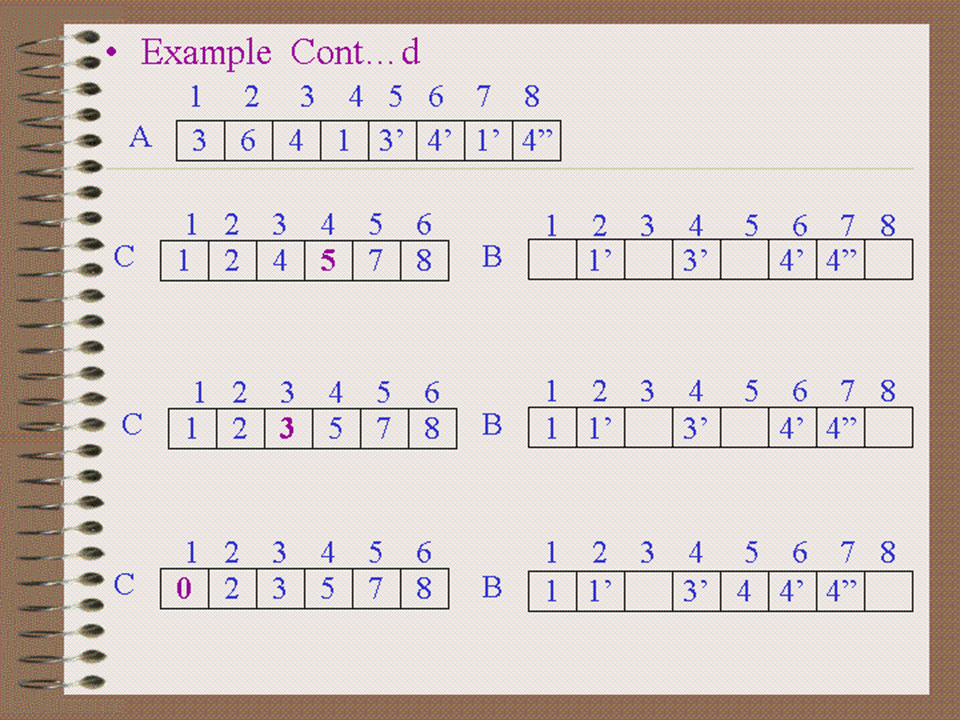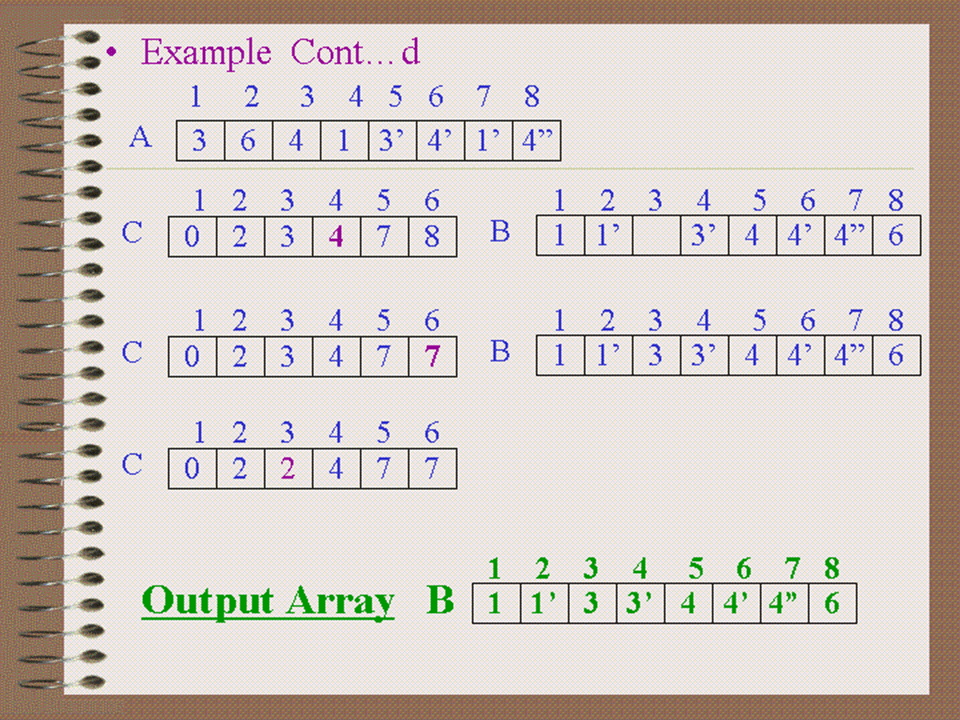# Counting sort is a type of Linear Time Sort

• Counting Sort was invented by H.H.Seward in 1954
• All the sorting algorithms introduced so far share an                                                interesting property: the sorted order they determine is based only on comparison between the input elements

## Assumption of Counting Sort

• Counting sort assumes that each of the element is an integer and lies in the range 1 to k, for some integer k
• When k=O(n) then sort runs in O(n) time.
• Determine how many elements are less than an element x
• Then place x directly on its correct position## Running Time

• T(n) = O(k) + O(n) + O(k) + O(n)

= O(k+n+k+n) = O(2k + 2n)

Assumption: k is O(n)

Hence T(n) = O(n)

Thus Counting Sort beats the running time of “nlogn” as it is not a comparison based sort